# NCERT Solutions for Class 9 Maths Exercise 7.5## myCBSEguide App

CBSE, NCERT, JEE Main, NEET-UG, NDA, Exam Papers, Question Bank, NCERT Solutions, Exemplars, Revision Notes, Free Videos, MCQ Tests & more.

NCERT Solutions for Class 9 Maths Exercise 7.5 book solutions are available in PDF format for free download. These ncert book chapter wise questions and answers are very helpful for CBSE board exam. CBSE recommends NCERT books and most of the questions in CBSE exam are asked from NCERT text books. Class 9 Maths chapter wise NCERT solution for Maths Book for all the chapters can be downloaded from our website and myCBSEguide mobile app for free.

NCERT solutions for Class 9 Maths Triangles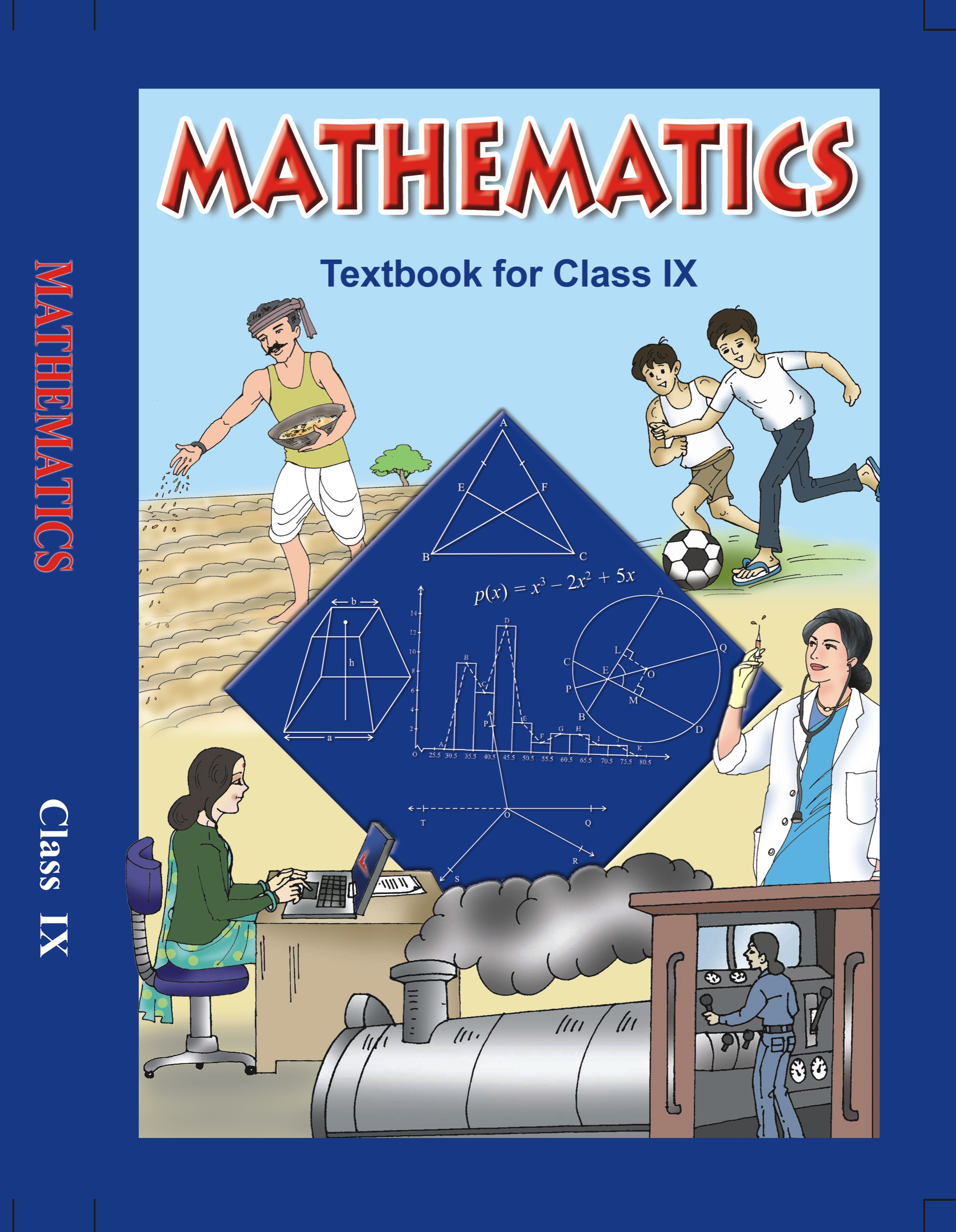## NCERT Solutions for Class 9 Mathematics Triangles

###### 1. ABC is a triangle. Locate a point in the interior of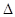ABC which is equidistant from all the vertices ofABC.

Ans. Let ABC be a triangle.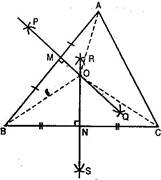Draw perpendicular bisectors PQ and RS of sides AB and BC respectively of triangle ABC. Let PQ bisects AB at M and RS bisects BC at point N.

Let PQ and RS intersect at point O.

Join OA, OB and OC.

Now inAOM andBOM,

AM = MB [By construction]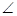AMO =BMO =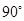[By construction]

OM = OM [Common]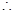AOM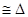BOM [By SAS congruency]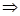OA = OB [By C.P.C.T.] …..(i)

Similarly,BONCONOB = OC [By C.P.C.T.] …..(ii)

From eq. (i) and (ii),

OA = OB = OC

Hence O, the point of intersection of perpendicular bisectors of any two sides ofABC equidistant from its vertices.

NCERT Solutions for Class 9 Maths Exercise 7.5

###### 2. In a triangle locate a point in its interior which is equidistant from all the sides of the triangle.

Ans. Let ABC be a triangle.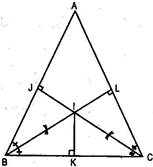Draw bisectors ofB andC.

Let these angle bisectors intersect each other at point I.

Draw IK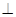BC

Also draw IJAB and ILAC.

Join AI.

###### InBIK andBIJ,IKB =IJB =[By construction]IBK =IBJ

[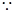BI is the bisector ofB (By construction)]

BI = BI [Common]BIKBIJ [ASA criteria of congruency]IK = IJ [By C.P.C.T.] ……….(i)

Similarly,CIKCILIK = IL [By C.P.C.T.] ……….(ii)

From eq (i) and (ii),

IK = IJ = IL

Hence, I is the point of intersection of angle bisectors of any two angles ofABC equidistant from its sides.

NCERT Solutions for Class 9 Maths Exercise 7.5

###### 3. In a huge park, people are concentrated at three points (See figure).

A: where there are different slides and swings for children.

B: near which a man-made lake is situated.

C: which is near to a large parking and exit.

Where should an ice cream parlour be set up so that maximum number of persons can approach it?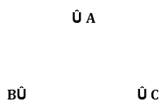Ans. The parlour should be equidistant from A, B and C.

For this let we draw perpendicular bisector say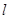of line joining points B and C also draw perpendicular bisector say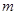of line joining points A and C.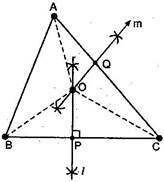Letandintersect each other at point O.

###### Now point O is equidistant from points A, B and C.

Join OA, OB and OC.

Proof: InBOP andCOP,

OP = OP [Common]OPB =OPC =BP = PC [P is the mid-point of BC]BOPCOP [By SAS congruency]OB = OC [By C.P.C.T.] …..(i)

Similarly,AOQCOQOA = OC [By C.P.C.T.] …..(ii)

From eq. (i) and (ii),

OA = OB = OC

Therefore, ice cream parlour should be set up at point O, the point of intersection of perpendicular bisectors of any two sides out of three formed by joining these points.

NCERT Solutions for Class 9 Maths Exercise 7.5

###### 4. Complete the hexagonal rangoli and the star rangolies (See figure) but filling them with as many equilateral triangles of side 1 cm as you can. Count the number of triangles in each case. Which has more triangles?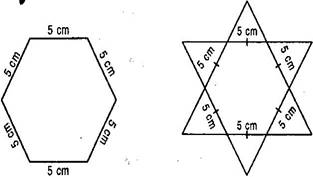Ans. In hexagonal rangoli, Number of equilateral triangles each of side 5 cm are 6.

Area of equilateral triangle =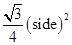=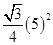=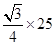sq. cm

###### Area of hexagonal rangoli = 6 x Area of an equilateral triangle

=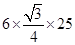=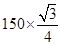sq. cm ……….(i)

Now area of equilateral triangle of side 1 cm = ==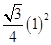=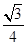sq. cm …..(ii)

Number of equilateral triangles each of side 1 cm in hexagonal rangoli

=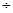=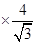= 150 …..(iii)

Now in Star rangoli,

Number of equilateral triangles each of side 5 cm = 12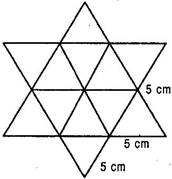###### Therefore, total area of star rangoli = 12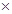Area of an equilateral triangle of side 5 cm

=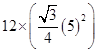=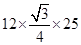=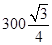sq. cm ……….(iv)

Number of equilateral triangles each of side 1 cm in star rangoli

=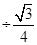== 300 ……….(v)

From eq. (iii) and (v), we observe that star rangoli has more equilateral triangles each of side 1 cm.

## NCERT Solutions for Class 9 Maths Exercise 7.5

NCERT Solutions for Class 9 Maths PDF (Download) Free from myCBSEguide app and myCBSEguide website. Ncert solution class 9 Maths includes text book solutions from Mathematics Book. NCERT Solutions for CBSE Class 9 Maths have total 15 chapters. 9 Maths NCERT Solutions in PDF for free Download on our website. Ncert Maths class 9 solutions PDF and Maths ncert class 9 PDF solutions with latest modifications and as per the latest CBSE syllabus are only available in myCBSEguide.

## CBSE app for Class 9

To download NCERT Solutions for Class 9 Maths, Computer Science, Home Science,Hindi ,English, Social Science do check myCBSEguide app or website. myCBSEguide provides sample papers with solution, test papers for chapter-wise practice, NCERT solutions, NCERT Exemplar solutions, quick revision notes for ready reference, CBSE guess papers and CBSE important question papers. Sample Paper all are made available through the best app for CBSE students and myCBSEguide website.### 1 thought on “NCERT Solutions for Class 9 Maths Exercise 7.5”

1. It’s very useful where we are confused…it’s also descriptive and helpful to gain Mark’s